Fold

The basic example of Fold is given below, but first I clear values from all variables.

ClearAll["Global`*"];
Fold[f,x,{a,b,c}]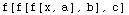The basic example above doesn't do much to help one understand how to make a useful program using Fold.  Some more illuminating examples are given below.  If you don't understand the #& notation see the discussion of Function. The three examples below aren't very useful, but they demonstrate the form that is normally needed when using Fold.

From these examples we get:
((((a+b)+c)+d)+e)

((((a b)c)d)e)

StringJoin[StringJoin[StringJoin[StringJoin[StringJoin[
"","a"],"b"],"c"],"d"],"e"]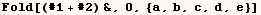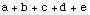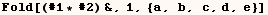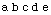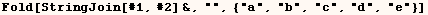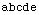A more useful application of Fold is given in the next cell.  Here we have a function that expresses a polynomial using Horner's rule.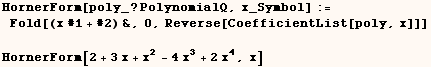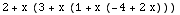In the Cell below Fold is used to make an Alternating Series.  If ( lst ) was used instead of (Reverse[lst] ),  the first term would be multiplied by (-1) when the Length of ( lst ) is even.  Jerry Keiper posted this solution to the MathGroup.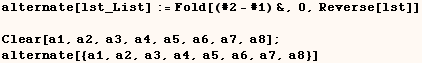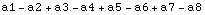Another application of Fold is shown below (copied from the Help Browser).  This function (EvaluateAt) takes an expression and a list of positions, and evaluates in place only the parts at the specified positions.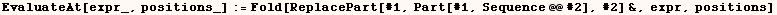To demonstrate this function consider the expression below which is wrapped in HoldForm to prevent evaluation.First we use ReleaseHold to see what the expression evaluates to without HoldForm.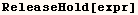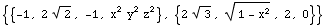In the next cell EvaluateAt (defined above) is used to evaluate only the parts of (expr) that contain trigonometric functions.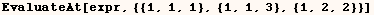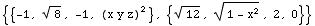If you have trouble figuring out the position of a portion of a sub-expression you are interested in you might want to down load a package Called (ExpressionManipulation.m) from http://home.earthlink.net/~djmp/Mathematica.html .

An alternate method of getting the same result in this example is given in the discussion of Block.

For another elegant application of Fold see the program Alan Hayes wrote which quickly finds the integers Relatively Prime to an integer n using Fold.

Created by Mathematica  (May 16, 2004)

Back to Ted’s Tricks index page